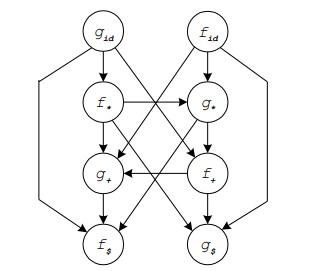# Explain Operator grammar and precedence parser in TOC

Data Structure AlgorithmsComputer ScienceComputers

If the grammar satisfies the following two conditions, then we can say that type of grammar is called as operator precedence grammar.

• If ε is on its RHS then there exists no production rule.

• If two non-terminals are adjacent to each other on its RHS then there exists no production rule.

Operator Grammars have the property that no production right side is empty or has two adjacent non-terminals.

## Example

E-> E A E | id

A-> + | *

The above grammar is not an operator grammar but we can convert that grammar into operator grammar like −

E-> E + E | E * E | id

There are three precedence relations, which are given below −

Relation
Meaning
ab
a yields precedence to b
a = · b
a has the same precedence as b
ab
atakes precedence over b

Precedence Table

id
+
*
$id + *$

Precedencce Table

## Example

The input string is as follows −

id1 + id2 * id3

After inserting precedence relations is−

$<· id1 ·> + <· id2 ·> * <· id3 ·>$

## Basic Principle

• Scan the string from left until seeing ·> and put a pointer.

• Scan backwards the string from right to left until seeing <·

• Everything between the two relations <· and ·> forms the handle.

• Replace handle with the head of the production.

## Operator Precedence Parsing Algorithm

The algorithm is as follows −

Initialize: Set P to point to the first symbol of the input string w$Repeat − Let b be the top stack symbol, a is the input symbol pointed to by P. if (a is$ and b is $) return else if a ·> b or a =· b then push a onto the stack advance P to the next input symbol else if a <· b then repeat c -> pop the stack until (c .> stack-top) else error end Example id + *$
id

+

*

$Construct a graph using the algorithm. This graph is as follows −By seeing this, we can extract the precedence function like − id + *$
f
4
2
4
0
g
5
1
3
0